# Binary Conversion For Dummies! (To Hex & Dec)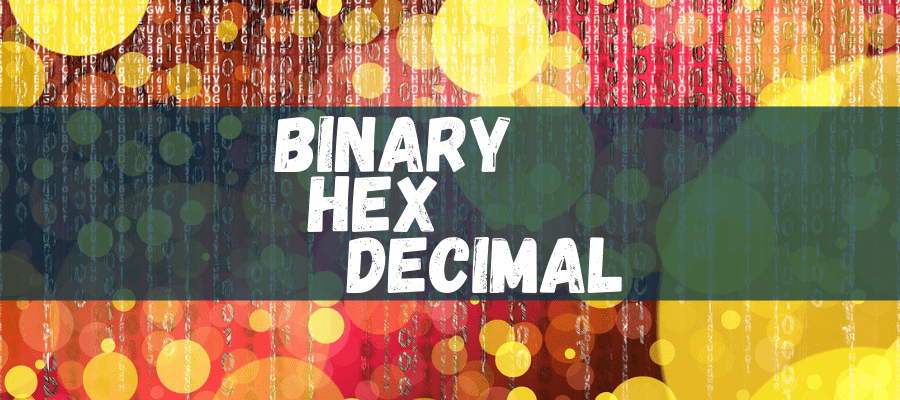3.3
(3)

Are you ready to learn binary conversion for dummies?!

This post will teach you how to convert the binary, hexadecimal and decimal numbering systems in a simple to learn format without all the complex math.

My aim here is to convince you that it really is very simple.. trust me!

Have you made it this far? good! I don’t want you to think that you’re about to learn something hard because it really isn’t.

This is really basic maths. I was never great at mathematics at school and I’m still not great at it now.

But what I do know is that 1 plus 2 is equal to 3.

This is what we’re really dealing with here.

Are you ready to learn your new found skills and fundamental knowledge on how computers work? lets go!

## 1. Background

### Information vs Data

We all know (or should do) that all computers need to store and send information.

That’s how communication works right?

Well, First off I need to correct what I have just said!

It’s in fact data that is stored and not information.

What is the difference between data and information you may be asking..

Well, information makes sense when you are given it. For example: I say the sentence “My name is installtekz”. This is information.

However if I say to you “*—*–*-*–” then you have no idea what this means, but you could interpret it in to something meaningful if we agree on what the characters mean.

We can only transmit and receive numbers across the network so we need to take all of our keyboard characters and give them each a unique number.

### It's All Just One's And zero's..! But What Are They?

If you ask anyone who works in IT just how data is stored then they will most likely tell you that it’s stored in one’s and zero’s.

But how on earth can a number be stored like this?

If you ask an Electronics Engineer how data is stored electronically then they will probably give a different answer.

The problem here is that IT professionals stay at the computer level and there’s no overlap in the knowledge to go deeper in to the electronics.

### It's All Magnetic

Most of us know that a magnet has two poles.. a north pole and a south pole. A bit (binary digit) is the basic building block of data.

Now if I was to go even deeper than electronic here I would be getting into quantum mechanics but I have zero knowledge on this subject so this is where I will be explaining from.

A bit (magnet) can have either a north pole or a south pole. When a computer writes data it is giving the bit either a north polarity or a south polarity.

The way it does this is by using two different voltages. These could be 0.7volts for a “0” and +5volts for a “1” for example.

### ASCII

We can only send numbers over a network so what we needed to do was assign each keyboard character it’s own number.

The ‘American Standard Code for Information Interchange’ is a character encoding system that maps our keyboard characters to a pre-defined number.

Just note that when I use a number for a character in this article that I have not just made a number up, but I have taken the relevant number from this ASCII character set.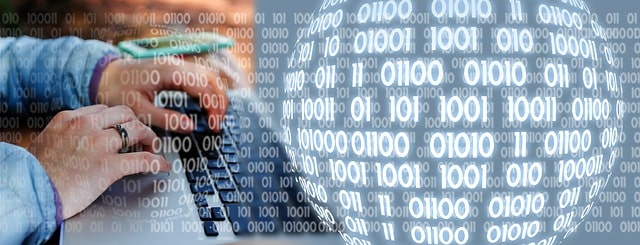## 2. What Is Decimal?

Do you know how to count to 10? Do you know how to count to 100?

Yes? That’s it. You already know decimal! Let’s move along. 🙂

Okay joking aside. Decimal is what we call a Base 10 numbering system.

This is the numbering system that we all learn as a child.

As this has always been the numbering system that we have ever known, it’s quite hard to wrap your head around the fact that a different numbering system can be used.

No one knows why humans use 10 as the base of our numbering system but the most obvious reason would be because we have have 10 fingers.

We used to use our fingers to help us count when we were young (yes I know, some of us still do) but I think we would find it hard to learn a base 11 numbering system if we only had 10 fingers right?

I won’t lie to you. All we have ever known is the base 10 numbering system. So understanding what another numbering system exists is like understanding a parallel universe or another dimension.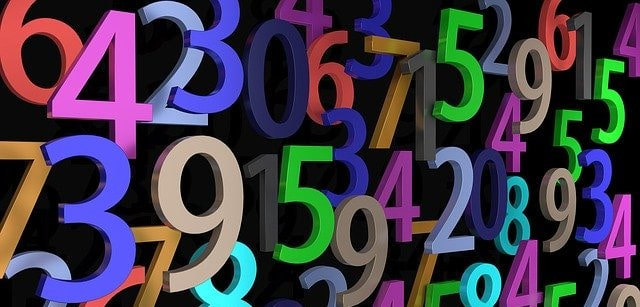## 3. What Is Binary And How Does It Work?

It’s all just bits and bytes.

Bit meaning BInary digiT, byte meaning BinarY digiT Eight.

Eight bits make up one byte. If we split a byte in two right down the middle then we have what’s called a nibble.

Binary is what we call a base 2 numbering system.

That’s because the total numbers we can use is two and they consist of either a 1 or a 0.

(our decimal numbering system has 10, which are zero through to nine.)

For the rest of this explanation I’m going to lay out one byte so we have a row of eight bits all together side-by-side.

We will be multiplying numbers here and the bit on the far left will have the highest multiplier so we call this the most significant bit.

The bit on the far right has the smallest multiplier so we call this the least significant bit.

We need to memorise something here but I promise it’s not too difficult.

The multiplier on the left (the most significant bit) has a multiplier of 128.

What we need to do though is start from the other end. Always start from the least significant bit when ever working with binary here.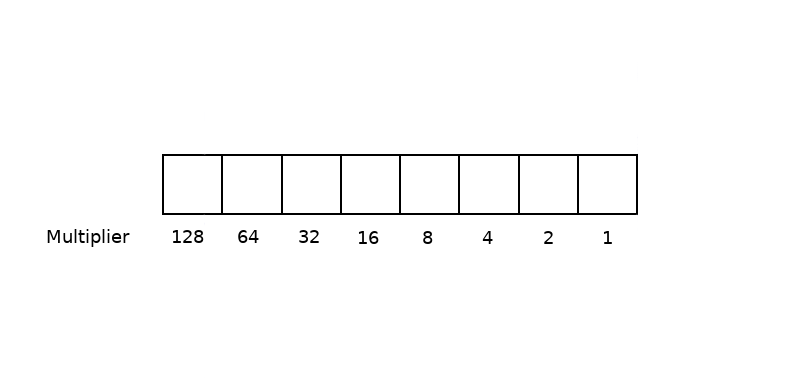The above image shows the multiplier numbers.

We need to memorise these numbers but if you look closely it isn’t difficult.

All we need to do is start on the right hand side, start from 1, and double that number seven times.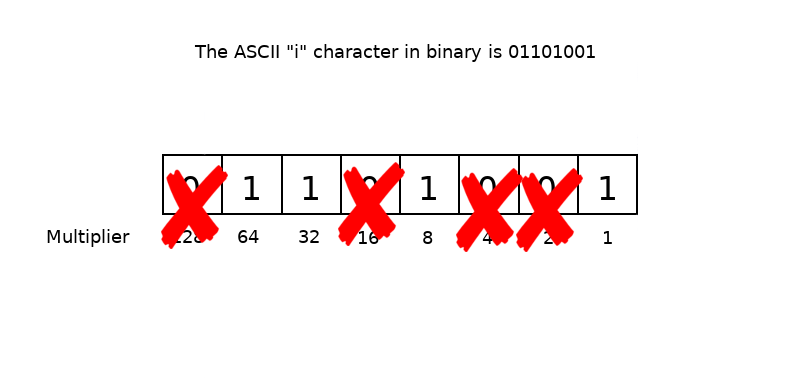Now, we just ignore the multiplier numbers that are below any zero’s.

Then we just add up all the remaining numbers. So, 64 + 32 + 8 + 1 = 105.

So the decimal value of “i” is 105. Congratulations! you have just converted binary to decimal.

## 4. What Is Hexadecimal (Hex) And How Does It Work?

Now we move to hexadecimal and I promise it’s just as easy and you’re already most of the way by now anyway if you have been following along.

Hexadecimal is a base-16 numbering system.

That is, we use sixteen different numbers and they are zero through to nine (0-9) and letters “a” through to “f”.

It’s important to note that the letters can be either uppercase or lowercase as they both can be used interchangeably and they mean the exact same number.

Now all we need to do is split our byte into two.

We then treat these two nibbles as if they were mini bytes. So now our multiplier only reaches 8 (for each nibble).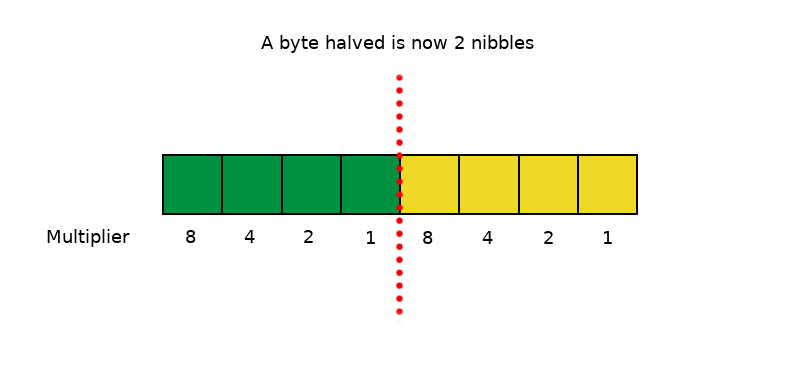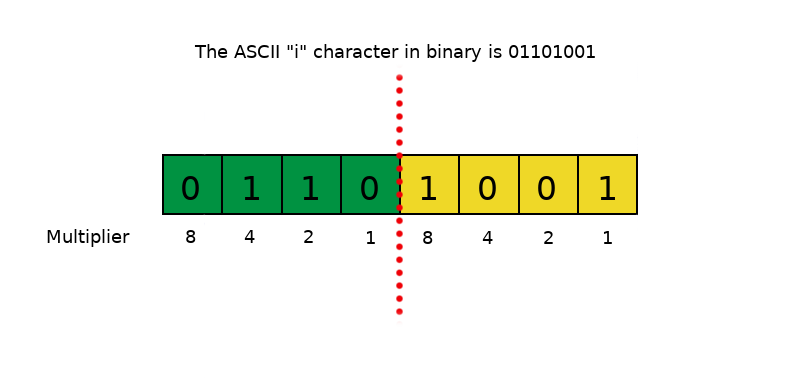Next we place our binary representation of the character “i” into our byte as we did before.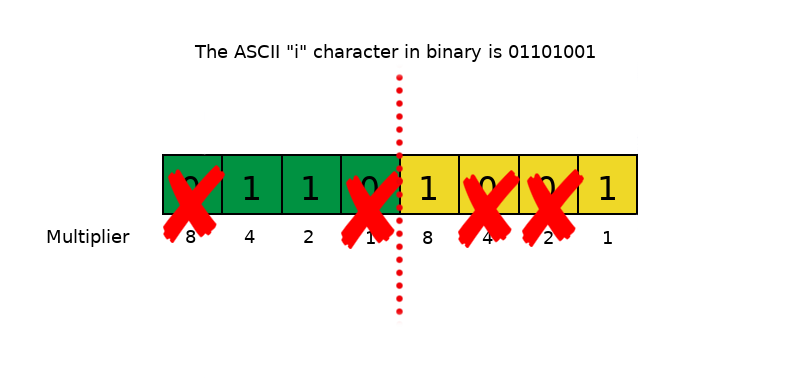We ignore any multiplier that’s directly below any zero’s (like we did before).

Now we will have two numbers. 4 + 2 = 6, and 8 + 1 = 9. So, the hexadecimal representation of the character “i” is 69.

Congratulations! You have now converted binary to hexadecimal!If we head on over to LookupTables.com we can find out what characters are assigned in various numbering systems, such as Decimal, Hexadecimal and Octal.

We won’t be dealing with Octal in this tutorial but for now, all you need to know is that is just another numbering system.

Octal is base 8. It uses numbers zero through to 7 (0-7) to represent it’s digits.

As you can see in the image above, I have highlighted in green the “i” (lowercase i) character.

The decimal representation for the character “i” is 105. The hex representation for “i” is 69.

## 5. Convert FROM Decimal TO Binary

Now that we have taken a binary number and converted it to both a hex number and a decimal number, let’s take a decimal number and convert it to a binary.I’m going to start by taking the above image of our byte multiplier and I’m going to select a random character from the ASCII table from further up this post.

I’m going to select the uppercase “T” character.

We can see from the chart the “T” in decimal is “84”.

Now what we do, is start from the LEFT hand side (the MOST significant bit) this time on our multiplier image.

Then I ask myself “Does 128 fit in to 84?” That answer is NO. So we place a zero in the 128 box.

The I ask myself “Does 64 fit in to 84?” and that answer is YES. So I’m now going to place a “1” inside the box above the “64” multiplier.

Now I make a mental note that we have “20” remaining. Next I ask myself “does 32 fit inside 20?” That answer is NO, so I place a zero above the “32”.

Next, “does 16 fit inside 20?” YES it does, leaving a remainder of “4”.

Then “8” will be a zero because it’s a higher number than 4.

4 now fits exactly in to 4 so we place a “1” in the box above the 4 multiplier.

We don’t have any remainder now so we place a zero in the boxes above the “2” and the “1”.

That’s it! our binary representation of the decimal value of “84” is 01010100.

## 6. Convert FROM Hex TO Binary And Decimal

The hex value of our uppercase “T” is 54.

We need to use our two nibbles now for this conversion as the “5” will be placed in the first four boxes on the left (the green boxes), and the “4” will be placed in the four boxes on the right (the yellow boxes).Next we need to add up the multiplier numbers so that we get the number “5”, and do the same to get the number “4”. As shown below: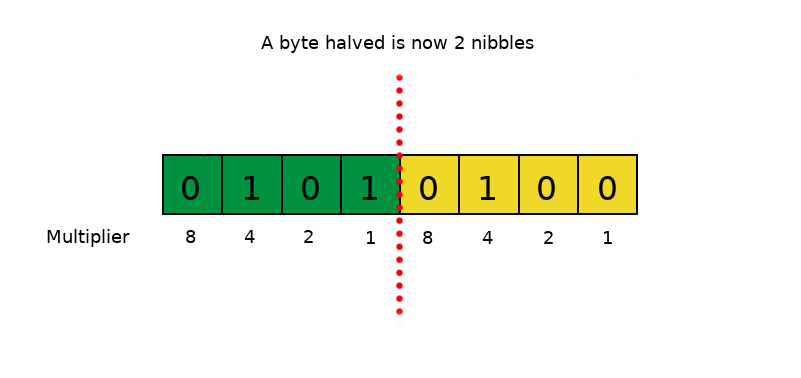That’s it. Simple isn’t it?

I’m going to go over another conversion but this time I’m going to use a hex number that uses letters for numbers.

It’s exactly the same thing, just remember that “a” or “A” is equal to “10”, “b” or “B” is equal to 11 and so on..

but only to “F” (F=15). Don’t forget that it’s also case-insensitive, meaning whether it’s uppercase or lowercase it’s exactly the same.

This time I’m going to use the lowercase “n” ASCII character. So looking at the chart above we can see that “n” in hexadecimal is “6E”. “E” is equal to “14”.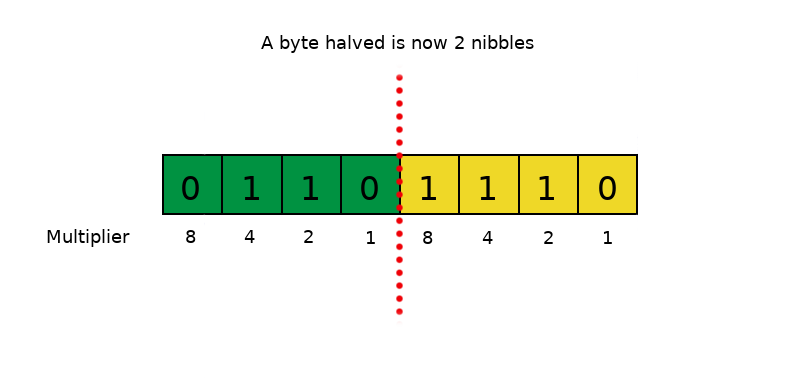4 + 2 = 6, and 8 + 4 + 2 = 14. So the binary representation of the ASCII character “n” is equal to “01101110” and the hex representation is “6E”.

If we wanted to get the decimal value of “n” we would need to use the byte multiplier below. this would be: 64 + 32 + 8 + 4 + 2 = 110!## Conclusion

And that’s it!

I told you it was easy.

After reading this post on ‘binary conversion for dummies’ you should hopefully know by now how to convert binary, hexadecimal and decimal numbers.

Just keep practicing!

This truly is a great skill to have and is the foundation of everything in our digital world.

After mastering this simple skill you can then go on to mastering IP addresses and much more!

IP addressing is part of TCP/IP networking and I have a post on this subject too. Why not give TCP/IP a try?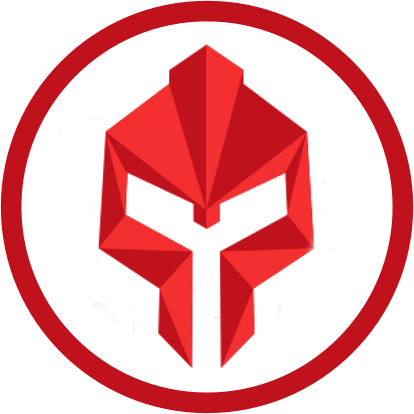#### installtekz

"When wireless is perfectly applied, the whole earth will be converted into a huge brain..." - Nikola Tesla, 1926